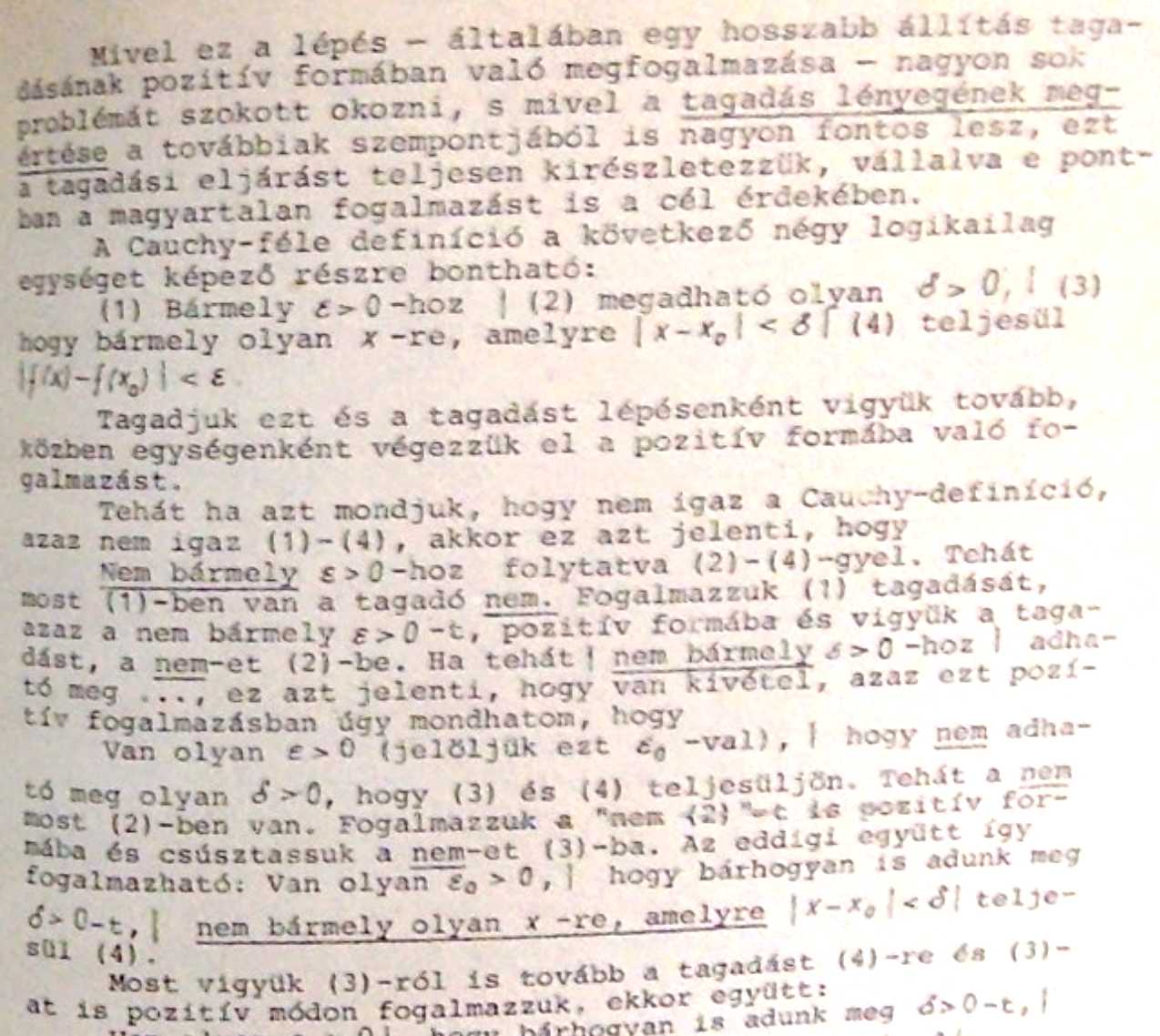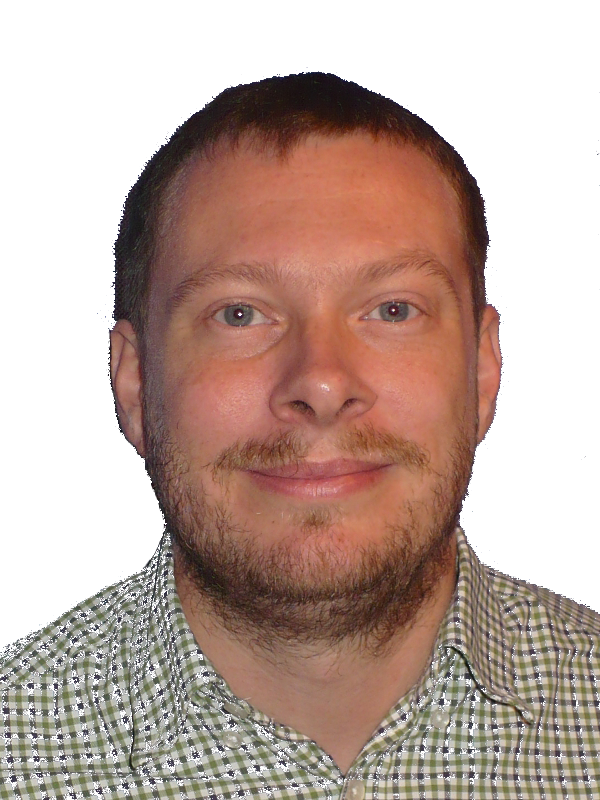# 2 November 2021

## Thoughts on supporting logic in function calculus with technology

These ideas began to come to my mind during the years, and had crystallized since I work on integrating Tarski into GeoGebra. As a start, consider the command RealQuantifierElimination(∃a a>0). Just copy-paste it in the CAS View below:

This is something completely new in GeoGebra, and the name of the command explains what theory is behind. In fact, here we do nothing else than proving, so maybe the command name Prove could be even better. Real quantifier elimination can give, of course, not just true or false outputs, but something more complicated. For example, RealQuantifierElimination(∃a a^2=b) returns $$b\geq0$$, so the statement $$\exists a:a^2=b$$ is true if and only if $$b$$ is non-negative. So, at the end of the day, this is quite similar how the ProveDetails command actually works. This question should be discussed with some GeoGebra educator experts soon.

On the other hand, entering a quantified formula is quite easy. I have not added the quantifiers $$\exists$$ and $$\forall$$ to any virtual keyboards yet (this should be rather discussed with the GeoGebra Team first), but the newly introduces keystrokes Alt-X and Alt-V are quite nice and convenient. Also, all former editing conveniences of GeoGebra can still be used, that is, for example, the implication sign $$\to$$ can be quickly accessed by typing “->” (a minus sign and a greater-than sign). A simple test input like RealQuantifierElimination(∀a a>1 -> a>0) shows this feature.

Here we arrived to one of the main benefits of this new technology. Learners can get some feedback if their mathematical input is correct or not, at least for a computer. Learning mathematics at school is usually not exact or precise enough in the strict mathematical sense, and this can harden understanding of some delicate concepts like convergence. At Johannes Kepler University, Linz, Austria, we usually have this problem with first-year mathematics teacher students. “Is my notation correct? This professor uses this notation, but another one uses something slightly different!” And this needs to be addressed somehow. Of course, many different notations can make sense, and the literature is not uniform in this question; but, actually, students should have a broader overview of mathematics than knowing only one notation for just one course. To be honest, some traditionally used notations can still be non-exact or even erroneous!

So I am biased towards uniformization, and this is what GeoGebra actually does, when you enter your input. It tries to identify what you mean, but then the input will be rewritten in some standard form, and displayed in the standardized variant. GeoGebra may however behave differently on different language settings, but for the topic of logic I am sure we can negotiate over some good standards, even if there are rather a lot of different standards out there, a couple of years after the computer based prover boom. The software tools Coq, Isabelle, LEAN, and many other rising stars search for new paths and exploit the power of technology even more and more, so it is not surprising that even the standards are still improving, every day!

Luckily, when implementing quantifiers in GeoGebra, I had very little choice on how to do it. Expression handling has a mature way in GeoGebra, and what I added yesterday was just extending an expression by a prefix $$\exists v$$ or $$\forall v$$ where $$v$$ is a variable. And nothing more. This automatically resulted in a very simple way of handling quantified expressions, and in some cases a couple of parentheses can also be spared by using the auto-simplification feature of GeoGebra. But if you accidentally get an error message that something is wrong with your input, just add some parentheses or spaces and give it another try.

### Some logic and calculus

I can still recall my university professor's favorite question during oral exams, back in the 1990s in Hungary. I think he was either professor Leindler, or one of his students, but the focus was on denying the definition of convergence. Anyway, his textbook was widely used among many generations of prospective math teachers. An important part of his book focuses on explicitly explaining how denying a quantifier statement should look like. This was extremely helpful for me in my further studies in calculus, and, actually, one cannot avoid such fine details when a precise proof is to be created. The figure below shows page 59 of the 3rd (unchanged) edition of this book from 1993 (in Hungarian): it is exactly about how the detailed negation of a quantified expression should look like.One may warn that this is too much for the classroom, but let me tell you: no, not at all! Precise definition of convergence is present in many secondary school textbooks in Austria, even if many students (and maybe several teachers) have no idea what it is about. This copy is taken from the book Mathematik verstehen (Understanding mathematics) for 16 years-old learners, and even if this is just a marginal part of the textbook, it is still there. (See page 134.)There are some difficulties with this formulation, and actually, the latest improvements in GeoGebra do not support it at all. On the other hand, the concept of infinite sequences is much more difficult than one can think of. Some of my experiences in private tutoring in Austria confirms this: the concept of choosing infinitely many numbers (and keeping them in mind at the same time) is not a trivial idea. The misconception that just a finite amount of numbers designates a sequence will not lead to the concept of convergence. This is quite remarkable because the topics of difference quotient and differential quotient are unremovable parts of the Austrian curriculum, and without a clear notion of convergence they have no clear meaning at all.

That is, instead of using the concept of infinite sequences we may have more luck with the concept of limit of a function. First of all, designation of infinitely many points by drawing a continuous curve is easily possible, already in kindergarten. On the other hand, we can focus on the definition via $$\varepsilon$$ and $$\delta$$, and by using this alternative way given by Cauchy we will exploit the benefits of real quantifier elimination. (The traditional definition via sequences was introduced by Heine.)

For a quite difficult example we can consider the function $$y=x^2$$ at infinity. It diverges to infinity there. In mathematical notation, $\lim_{x\to\infty} x^2=\infty,$ but this is, of course far from being trivial for a student. One difficulty is that $$\infty$$ is presented twice in the formula, and another one is that the notion of convergence of a sequence is implicitly mentioned under the $$\lim$$: the notation cannot help us forgetting the definition à la Heine. Also, it is not straightforward to translate this formula into a quantified one à la Cauchy, but this is how it looks like: RealQuantifierElimination(∀M (∃N (∀X (X > N → X² > M)))). In fact, M stands for the “right” $$\infty$$ and N for the “left” one. From a technical aspect here we note that the current version of GeoGebra Discovery disallows using lowercase x, but this issue will be addressed hopefully soon.

### Introductory examples

In a classroom I would not start using this new piece of technology without telling the basics. So, instead of jumping into deep water I'd begin with some simple definitions. Also, finding the negation of a statement could be extremely helpful; this is, however, not yet supported in GeoGebra Discovery. For the future I plan using Tarski's t-neg command which just negates the quantified input expression.
1. Simple properties of real numbers. For example: there is no greatest real number (RealQuantifierElimination(∀a (∃b b>a)), or its negation RealQuantifierElimination(∃a (∀b b≤a))).
2. Simple properties of real functions. E.g.: the function $$y=x^3$$ is monotonically increasing (RealQuantifierElimination(∀a (∀b a<b → a^3<b^3))). Here we need to admit that only algebraic functions are supported, that is, for example, exponential or trigonometric function cannot be used. Also, a technical issue that division may need to be worked around by multiplications. Another simple example is boundedness: the function $$y=x^2+2$$ is not bounded: RealQuantifierElimination(∀M (∃a a^2+2>M)).

### Some more difficult examples

1. Convergence at point. E.g.: the function $$y=x^2$$ has the limit 9 at $$x=3$$: RealQuantifierElimination(∀E E>0 → (∃D D>0 ∧ (∀X ((X-3<D ∧ X-3>-D ∧ X!=3) → (X^2-9 < E ∧ X^2-9 > -E))))). (It would be nice to use the absolute value instead of writing the complicated conjunction.) We can find this limit by writing A instead of 9: RealQuantifierElimination(∀E E>0 → (∃D D>0 ∧ (∀X ((X-3<D ∧ X-3>-D ∧ X!=3) → (X^2-A < E ∧ X^2-A > -E))))). Or, if we are not interested which A is associated, we can just write: RealQuantifierElimination(∃A (∀E E>0 → (∃D D>0 ∧ (∀X ((X-3<D ∧ X-3>-D ∧ X!=3) → (X^2-A < E ∧ X^2-A > -E)))))). Note that it is usually not trivial for the students (and teacher, either) how to use this linear definition, especially when a conjunction or an implication needs to be used.
2. Continuity. E.g.: the function $$y=x^2$$ is continuous: RealQuantifierElimination(∀X0 (∀E E>0 → (∃D D>0 ∧ (∀X ((X-X0<D ∧ X-X0>-D) → (X^2-X0^2 < E ∧ X^2-X0^2 > -E)))))). Clearly, here an improvement in allowing Greek characters ($$\varepsilon$$ and $$\delta$$ instead of E and D) or indexes ($$x_0$$ instead of X0) could increase the user experience.

### Is this something really new?

In fact, all of this is more or less well-known in the literature, but, because of lack of a suitable free software toolset, until now it was not really possible to exploit technology in the classroom in this direction. On some related ideas I suggest reading my joint paper with Peter Mayerhofer, moreover the PhD thesis written by Róbert Vajda (Supporting Exploration in Elementary Analysis by Computational, Graphical and Reasoning Tools, Johannes Kepler University, Linz, 2009).

To make this free toolset ready for classroom use, I think some additional technical steps are still required. First of all, quantified expressions, without calling them via the RealQuantifierElimination command, return just an undefined value in the CAS View. This should be somehow improved. Further ideas are to visualize the cylindrical algebraic decomposition if the number of variables is no more than three (there is already support for this in Tarski), or just to provide a truth table if there are no quantifiers present.

Anyway, the most important feature is already present and ready: The computer can give feedback if a conjecture is true or false. Is my concept on a mathematical definition, related to functions, correct? This can be tested quite quickly in a web browser. It is learning mathematics by doing it: this is what exactly we expect from our students, and now technology is very close to help them.

Either on this page or here you can try the new feature. Alternatively, you can download the approprate version of GeoGebra Discovery from its official repository.

### Acknowledgment

Jan-Michael Holzinger found a minor error in a former version of this entry. It is now fixed. Thanks, Jan!Zoltán Kovács Linzer Zentrum für Mathematik Didaktik Johannes Kepler Universität Altenberger Strasse 54 A-4040 Linz